# College Chemistry : Periodic Trends

## Example Questions

### Example Question #1 : Periodic Trends

Which of the following elements has the most metallic character: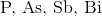?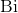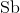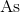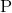Explanation:

The metallic character of elements increases as you move down a group. All of the listed elements are in Group 5. Sinceis the furthest down, it must have the most metallic character.

### Example Question #82 : Introductory Topics

Which of the following elements has the largest atomic radius: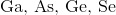?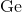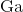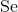Explanation:

When looking at a periodic table, you should notice that all of these elements are in the same period. Recall that atomic radii decrease as you move right on a period. Thus, this is the order of the elements by decreasing radius: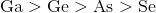, or gallium, has the largest radius because it is the furthest left on the periodic table.

### Example Question #11 : Atoms And Elements

The set of elements with the highest first ionization energies are known as which of the following?

Metalloids

Halogens

Noble gases

Alkali metals

Alkaline earth metals

Noble gases

Explanation:

The noble gases possess a full octet of electrons. They have the largest first ionization energies because of their tendency to keep all of their valence electrons. Halogens have the highest electron affinity; therefore, they have high—but not the highest—ionization energies. The alkali and alkaline earth metals have low electron affinities and low ionization energies. Last, the metalloids possess ionization energies that are neither high nor low.

### Example Question #84 : Introductory Topics

Rank the following in order of increasing metallic character: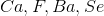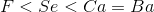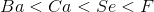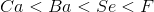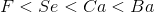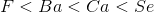Explanation:

The correct answer ishas the most nonmetallic characteristics while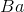has the most metallic characteristics. According to periodic trends, metallic character decreases from left to right across a period and increases top to bottom. Therefore,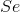will show less metallic character than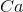.

### Example Question #272 : College Chemistry

Which of these atoms is the smallest?

Beryllium

Barium

Strontium

Calcium

Magnesium

Beryllium

Explanation:

The atomic radii is the size of an atom when it is not bonded to any other atoms. The periodic table can be used to estimate the size of the atomic radii of atoms. As you move down the periodic table the atomic radii increase, but as you move from left to right on the periodic table, the size of the atomic radii decrease.

Beryllium, magnesium, calcium, strontium, and barium are all in the same group on the periodic table, so the smallest element is the element closest to the top of the periodic table since the atoms become larger as you go down the periodic table. Therefore, the smallest atom of this group is beryllium.

### Example Question #86 : Introductory Topics

Which atom is the most electronegative?

Aluminium

Silicon

Sulfur

Phosphorus

Chlorine

Chlorine

Explanation:

Electronegativity refers to the ability of an atom to attract and bind electrons.  If the valence electrons are less then half full, then it takes less energy to lose an electron than gain electron making these atoms less electronegative. If the valence electrons are more than half full, then it takes less energy to gain electrons compared to losing an electron; therefore these electrons are more electronegative. The periodic table can be used to predict the electronegativity of the atom and how it compares to other atoms. As you move left to right of the periodic table, the electronegativity increases because the atoms on the right side of the periodic table have more valence electrons, and smaller radii. As you move down the periodic table the electronegativity decreases because the atomic number increases resulting in a greater distance between valence electrons and the nucleus causing less pull on the valence electrons.

Using the electronegativity periodic trend, we can predict which of the atom's is most electronegative. Aluminium, silicon, phosphorous, sulfur, and chlorine are all in the same row in the periodic table. Therefore, chlorine is the most electronegative because it is the farthest right on the periodic table so it has the most valence electrons and it will cost less energy to gain an electron than lose an electron.

### Example Question #1 : Periodic Trends

Which of the following atoms in the largest?

Sulfur

Sodium

Aluminium

Phosphorous

Chlorine

Sodium

Explanation:

The atomic radii is the size of an atom when it is not bonded to any other atoms. The periodic table can be used to estimate the size of the atomic radii of atoms. As you move down the periodic table the atomic radii increase, but as you move from left to right on the periodic table, the size of the atomic radii decrease.

Sodium, aluminium, phosphorus, sulfur, and chlorine are all in the same row of the periodic table. Since the size of the atomic radii decreases as you move from left to right on the periodic table, the element furthest to the left will be the largest. Therefore, sodium is the largest atom out of the group.

### Example Question #88 : Introductory Topics

Which element is the least electronegative?

Oxygen

Polonium

Selenium

Tellurium

Sulfur